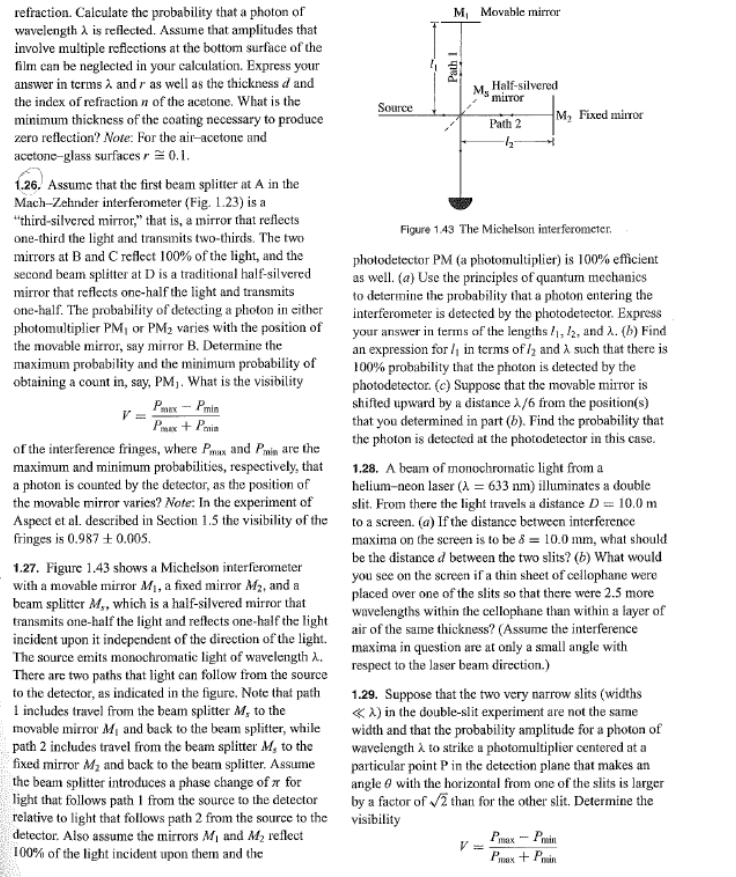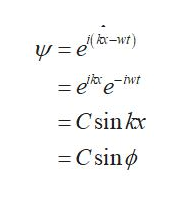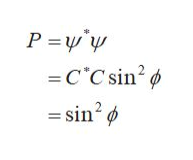refraction. Calculate the probability that a photon ofwavelength A is reflected. Assume that amplitudes thatinvolve multiple reficctions at the bottom surface of thefilm ean be neglected in your calculation. Express youranswer in terms A and r as well as the thickness d andM, Movable mirrorHalf-silveredMs'mirorthe index of refraction n of the acetone. What is theminimum thickness of the coating necessary to producezero reflection? Note: For the air-acetone andSourceM, Fixed mirrorPath 2acetone-glass surfaces r 0.11.26. Assume that the first beam splitter at A in theMach-Zehnder interferometer (Fig. 1.23) is a"third-silvered mirror," that is, a mirror that reflectsone-third the light and transmits two-thirds. The twomirrors at Band C reflect 100% of the light, and thesecond beam splitter at D is a traditional half-silveredmirror that reflects one-half the light and transmitsone-half. The probability of detecting a photon in eitherphotomultiplier PM, or PM2 varies with the position ofthe movable mirror, say mirror B. Determine themaximum probability and the minimum probability ofobtaining a count in, say, PM. What is the visibilityFigure 143 The Michelson interferometerphotodetector PM (a photomultiplier) is 100% efficientas well. (a) Use the principles of quantum mechanicsto determine the probability that a photon entering theinterferometer is detected by the photodetector. Expressyour answer in terms of the lengths , 12, and . (b) Findan expression for in terms of and A such that there is00 probability that the photon is detected by thephotodetector. (e) Suppose that the movable mirror isshifted upward by a distance /6 from the position(s)that you determined in part (b). Find the probability thatthe photon is detected at the photodetector in this case.PmaxPinPxPinof the interference fringes, where Pmax and Pmin are themaximum and minimum probabilities, respectively, thata photon is counted by the detector, as the position ofthe movable mirror varies? Note: In the experiment ofAspect et al. described in Section 1.5 the visibility of thefringes is 0.987 +0.0051.28. A beam of monochromatic light from ahelium-neon laser (A = 633 nm) illuminates a doubleslit. From there the light travels a distance D = 10.0 mto a screen. (a) Ifthe distance between interferencemaxima on the sereen is to be & = 10.0 mm, what shouldbe the distance d between the two slits? (b) What wouldyou see on the screen if a thin sheet of cellophane wereplaced over one of the slits so that there were 2.5 morewavelengths within the cellophane than within a layer of1.27. Figure 1.43 shows a Micheison interferometerwith a movable mirror M, a fixed mirror M2, and abeam splitter M,, which is a half-silvered mirror thattransmits one-half the light and reflects one-half the lightincident upon it independent of the direction of the light, air of the same thickness? (Assume the interferenceThe source emits monochromatic light of wavelength AThere are two paths that light can follow from the sourceto the detector, as indicated in the figure. Note that pathl includes travel from the beam splitter M, to themovable mirror M and back to the beam splitter, whilepath 2 includes travel from the beam splitter M, to thefixed mirror M2 and back to the beam splitter. Assumethe beam splitter introduces a phase change ofr forlight that follows path 1 from the source to the detectorrelative to light that follows path 2 from the source to thedetector. Also assume the mirrors Mi and M2 reflect100 % of the light incident upon them and themaxima in question are at only a small angle withrespect to the laser beam direction.)1.29. Suppose that the two very narrow slits (widthsA) in the double-slit experiment are not the samewidth and that the probability amplitude for a photon ofwavelength A to strike a photomultiplier centered at aparticular point Pin the detcction plane that makes anangle with the horizontal from one of the sits is largerby a factor of 2 than for the other slit. Determine thevisibilityPmax-PrinVPax+Pin

Question

I need help with this problem I got it wrong on my homework and wanted to know how to do it. The question is number 1.27 not the others.help_outlineImage Transcriptioncloserefraction. Calculate the probability that a photon of wavelength A is reflected. Assume that amplitudes that involve multiple reficctions at the bottom surface of the film ean be neglected in your calculation. Express your answer in terms A and r as well as the thickness d and M, Movable mirror Half-silvered Ms 'miror the index of refraction n of the acetone. What is the minimum thickness of the coating necessary to produce zero reflection? Note: For the air-acetone and Source M, Fixed mirror Path 2 acetone-glass surfaces r 0.1 1.26. Assume that the first beam splitter at A in the Mach-Zehnder interferometer (Fig. 1.23) is a "third-silvered mirror," that is, a mirror that reflects one-third the light and transmits two-thirds. The two mirrors at Band C reflect 100% of the light, and the second beam splitter at D is a traditional half-silvered mirror that reflects one-half the light and transmits one-half. The probability of detecting a photon in either photomultiplier PM, or PM2 varies with the position of the movable mirror, say mirror B. Determine the maximum probability and the minimum probability of obtaining a count in, say, PM. What is the visibility Figure 143 The Michelson interferometer photodetector PM (a photomultiplier) is 100% efficient as well. (a) Use the principles of quantum mechanics to determine the probability that a photon entering the interferometer is detected by the photodetector. Express your answer in terms of the lengths , 12, and . (b) Find an expression for in terms of and A such that there is 00 probability that the photon is detected by the photodetector. (e) Suppose that the movable mirror is shifted upward by a distance /6 from the position(s) that you determined in part (b). Find the probability that the photon is detected at the photodetector in this case. PmaxPin PxPin of the interference fringes, where Pmax and Pmin are the maximum and minimum probabilities, respectively, that a photon is counted by the detector, as the position of the movable mirror varies? Note: In the experiment of Aspect et al. described in Section 1.5 the visibility of the fringes is 0.987 +0.005 1.28. A beam of monochromatic light from a helium-neon laser (A = 633 nm) illuminates a double slit. From there the light travels a distance D = 10.0 m to a screen. (a) Ifthe distance between interference maxima on the sereen is to be & = 10.0 mm, what should be the distance d between the two slits? (b) What would you see on the screen if a thin sheet of cellophane were placed over one of the slits so that there were 2.5 more wavelengths within the cellophane than within a layer of 1.27. Figure 1.43 shows a Micheison interferometer with a movable mirror M, a fixed mirror M2, and a beam splitter M,, which is a half-silvered mirror that transmits one-half the light and reflects one-half the light incident upon it independent of the direction of the light, air of the same thickness? (Assume the interference The source emits monochromatic light of wavelength A There are two paths that light can follow from the source to the detector, as indicated in the figure. Note that path l includes travel from the beam splitter M, to the movable mirror M and back to the beam splitter, while path 2 includes travel from the beam splitter M, to the fixed mirror M2 and back to the beam splitter. Assume the beam splitter introduces a phase change ofr for light that follows path 1 from the source to the detector relative to light that follows path 2 from the source to the detector. Also assume the mirrors Mi and M2 reflect 100 % of the light incident upon them and the maxima in question are at only a small angle with respect to the laser beam direction.) 1.29. Suppose that the two very narrow slits (widths A) in the double-slit experiment are not the same width and that the probability amplitude for a photon of wavelength A to strike a photomultiplier centered at a particular point Pin the detcction plane that makes an angle with the horizontal from one of the sits is larger by a factor of 2 than for the other slit. Determine the visibility Pmax-Prin V Pax+Pin fullscreen
Step 1

Consider plane wave solution of a photon and write the expression fotr the wavefuntion.help_outlineImage Transcriptionclose(-w) ela-r) = eeht -Csin kx Csin fullscreen
Step 2

(a)

Write the expression for the probability of photon.help_outlineImage TranscriptioncloseP y - in2 - sin2 C C fullscreen
Step 3

The angle of the wave function is the phas...

Want to see the full answer?

See Solution

Want to see this answer and more?

Our solutions are written by experts, many with advanced degrees, and available 24/7

See Solution
Tagged in

Physics